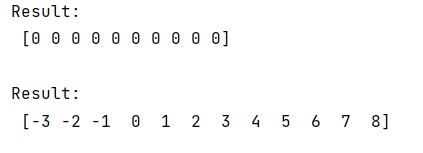# Module 'numpy' has no attribute 'arrange' (Fixed)

Learn, how to fix / solve the error - Module 'numpy' has no attribute 'arrange' (Fixed) in Python?
Submitted by Pranit Sharma, on February 20, 2023

NumPy is an abbreviated form of Numerical Python. It is used for different types of scientific operations in python. Numpy is a vast library in python which is used for almost every kind of scientific or mathematical operation. It is itself an array which is a collection of various methods and functions for processing the arrays.

## Solving the error "Module 'numpy' has no attribute 'arrange'"

Many times, programmers face this problem that they do not get the arange attribute of numpy and the only reason behind this is that they uses the word ‘arrange’ instead or ‘arange’ with a single ‘r'.

numpy.arange is used to return evenly spaced values within a given interval. arange can be called with a varying number of positional arguments:

• arange(stop): Values are generated within the half-open interval [0,stop) (in other words, the interval including start but excluding stop).
• arange(start,stop): Values are generated within the half-open interval [start, stop).
• arange(start,stop, step): Values are generated within the half-open interval [start, stop), with spacing between values given by step.

Let us understand with the help of an example,

## Python code to solve Module 'numpy' has no attribute 'arrange'

```# Import numpy
import numpy as np

# Using numpy.arange
res = np.arange(0, 5, 0.5, dtype=int)

# Display result
print("Result:\n",res,"\n")

# Using numpy.arange
res = np.arange(-3, 3, 0.5, dtype=int)

# Display result
print("Result:\n",res,"\n")
```

Output: